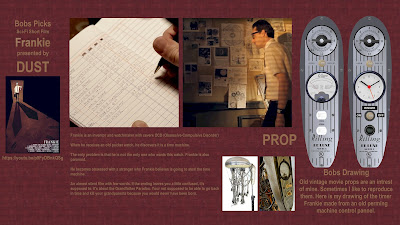## Tuesday, April 23, 2019

### TWO OUT OF EVERY FIVE AGREE...

TWO OUT OF EVERY FIVE AGREE...
Converting a binary number into a Decimal Number is confusing.
Seems simple enough.

16 8  4  2  1   <--- The weights of the bits in a Binary Coded Decimal number (BCD)
1  0  0  0  1    To find the decimal value just add up the weights of all the "1" bits.

So, to convert 10001 BCD to decimal, we just add 16 + 1 and get 17 decimal

To be more exact, we just converted a Binary Coded Decimal  (BCD) number to decimal.
However, BCD isn't the only way of coding a binary number.

Consider 2 of 5 binary numbers. The weight of each column is different from regular BCD.

6  3  2  1  0     The weights of the bits in a 2 of 5 coded binary number (2 of 5)
1  0  0  0  1      Again, we just add up the weights of the "1" bits.
So 6 + 0 = 6
In 2 of 5, we always have 5 bits and two of them are "1"s while the others are "0"s

Here is the complete list:

6 3 2 1 0 <--- The weights of the bits in a Binary Coded Decimal number (BCD)
0 0 0 1 1     1 + 0 = 1
0 0 1 0 1     2 + 0 = 2
0 1 0 0 1     3 + 0 = 3
0 1 0 1 0     3 + 1 = 4
0 1 1 0 0     3 + 2 = 5
1 0 0 0 1     6 + 0 = 6
1 0 0 1 0     6 + 1 = 7
1 0 1 0 0     6 + 2 = 8
1 1 0 0 0     6 + 3 = 9
0 0 1 1 0    2 + 1 =  0  (there are two ways to form "3", so this combination is taken to be zero.)

Notice that there is no way to combine two "1"s and three zeros to represent a decimal zero. Yet, there are two ways to convert a decimal 3 to two of five binary,

6 3 2 1 0  <--- The weights
0 1 0 0 1     3 + 0 = 3
0 0 1 1 0     2 + 1 =  3

### Alientologists

Future Paleontologist.

Alientologists
This has to be the only Science fiction  movie ever made that is also classified as a musical.

## Tuesday, April 2, 2019

### Movie Shorts on DUST - Frankie

My movie pick this week is Frankie)
Frankie is an inventor and watchmaker with severe OCD (Obsessive-Compulsive Disorder)
When he receives an old pocket watch, he discovers it is a time machine.

The only problem is that he is not the only one who wants this watch. Frankie is also paranoid.
He becomes obsessed with a stranger who Frankie believes is going to steal the time machine.

An almost silent film with few words. If the ending leaves you a little confused, it's supposed to. It's about the Grandfather Paradox. Your not supposed to be able to go back in time and kill your grandparents because you would never have been born.
Frankie is a "pure-bred" sci-fi adventure with retro elements. I love the timer Frankie made that is a modified part of an old hair curler.My wallpaper for Frankie.